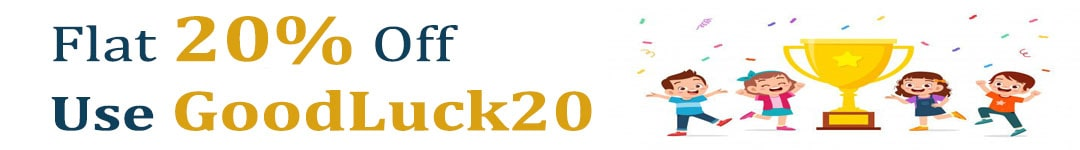International Maths Olympiad Forum By SOF Olympiad Trainer - Page 483

# User ForumSubject :IMO    Class : Class 4

Class : Class 4
C

## Ans 2:

Class : Class 5
Answer is C as the question says that 4 diamonds is 1240,we have to divide to get a diamond's value, which is 1240 divided by 4, the answer comes as 310. If we subtract a diamond from 1000, which is 1000-310, the answer comes as a square, which is 690. If we subtract a diamond from a

Class : Class 8
380

## Ans 4:

Class : Class 5
The answer is C as the question says that 4 diamonds is 1240 and 1240 divided by 4 is 310 and if we subtract a diamond from 1000 which means 1000-310,the answer comes as one square which is 690 and if we subtract a diamond from a square which means 690-310,the answer comes as 380.

## Ans 5:

Class : Class 4
Option C 380

Subject :IMO    Class : Class 3

## Ans 1:

Class : Class 4
No!

Subject :IMO    Class : Class 5

Class : Class 7
(B) 2.4 kg

## Ans 2:

Class : Class 6
Correct answer is 2.4kg

Subject :IMO    Class : Class 3

## Ans 1:

Class : Class 6
60 min

Subject :IMO    Class : Class 10

## Ans 1:

Class : Class 10
c. a-5b=0

Subject :IMO    Class : Class 7

## Ans 1:

Class : Class 7
Please explain this question

Class : Class 8

## Ans 3:

Class : Class 8
yeah.... i know... B should be the answer but it is telling that C is the answer

Class : Class 8
d is the answer

Class : Class 7
B is ans

Class : Class 7

## Ans 7:

Class : Class 7
yes exactly. b is ans. it says c is ans

Subject :IMO    Class : Class 3

## Ans 1:

Class : Class 3
Same here, also helpline no. 09953204707 is Switched Off.

Subject :IMO    Class : Class 3

Class : Class 3
A

Class : Class 1

Class : Class 2

Class : Class 4
A

Class : Class 7
it is A.

## Ans 6:

Class : Class 1
Yeah, But how can it be?

## Ans 7:

Class : Class 3
the correct and sure answer is A

Subject :IMO    Class : Class 3

Class : Class 6
D

Class : Class 4
D

Class : Class 5
D

## Ans 4:

Class : Class 3
d

Subject :IMO    Class : Class 7

## Ans 1:

Class : Class 7
The answer is option A

Class : Class 9
ans is option a

Class : Class 9